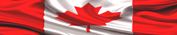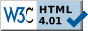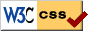HOME PAGE --------------- HELP+ » e-Design Notes --- » Switches, R, F » Resistors » Capacitors » Inductors » Diodes » Transistors --- » Potential Diff » Op Amps » Analog Filters --- » Boolean Algebra » Logic Gates » Flip-Flops » Logic ICs --- » Auto Injectors ---------------# ELECTRONIC DESIGN NOTES - INDUCTORS, TRANSFORMERSIn this page inductors and transformers are presented together, because they are closely related. The simplified structure employed to describe inductors/transformers is:

1. Electromagnetic Field
2. Electromagnetic Induction
3. Equivalent series and parallel inductors
4. Current growth/decay in inductive circuits
5. Transformers
6. Power Factor in AC circuits

NOTE

The basic notions highlighted in this page are related to a few electronic design topics presented in the first part, Hardware Design, of
LEARN HARDWARE FIRMWARE AND SOFTWARE DESIGN.1. ELECTROMAGNETIC FIELD

In addition to creating an Electrostatic Field, electric current flowing through conductors also creates an Electromagnetic Field--perfectly similar to the (mechanical) magnetic one, plus a few additional electrical characteristics.

Specific to both the magnetic and electromagnetic fields is, the "magnetic flux lines" are distinct (discrete): they go out from the conventional North Pole, then they enter the conventional South Pole. Therefore, in one particular point we can measure the number of magnetic field/flux lines (Φ) per unit of area (A), and that results in Magnetic Field Density (also known as the Electromagnetic Induction):

Magnetic Field Density:     B [tesla] = Φ [weber] / A [m2]

1 [T]  = 1 [Wb/m2] = 104 [G (Gauss--this is an older notation)]

Each conductor develops an electromagnetic field when electrical current passes through it. However, in order to amplify the electromagnetic field we need to coil our conductor a few times: the result is a solenoid having a number of turns (N), a length (L), and an inside coil area (A).

Note that inside our solenoid (the core) there is air, but we could easily introduce various metallic alloys instead. Now, different core materials have the quality of increasing, or of decreasing, the magnetic field density based on their characteristic Magnetic Permeability (μ).

Magnetic Permeability in vacuum (and also in air, because it is almost the same) is:

μo = 4*PI*10-7 [Wb/A*m]

A few formulas are of particular interest, therefore they are listed in the following table.

 ELECTROMAGNETIC FIELD FORMULAS Formulas Explanations B = μo*I / 2*PI*r Induction around a single straight conductor (wire) B = electromagnetic induction [T] μo = magnetic permeability in air l = the current through conductor in [A] r = distance of the reference point to the center of the conductor in [m] B = μo*I / 2*r Induction inside a single loop The sense of the B vector may be found using the right-hand rule r is radius of the loop in [m] in this case B = μo*N*I / 2*r Induction inside multiple (N) loops B = μo*N*I / L Induction at the center of a long solenoid L = the length of the solenoid Bm = χ*N*I / L Induction inside metallic cores χ is magnetic susceptibility B [Tesla] = Φ [Wb] / A [m2] B = Bo + Bm B = (μo*N*I / L) + (χ*N*I / L) B = μ * H B = (μo + χ) * (N*I / L) Magnetic Flux Density in metallic cores Bo  = magnetic flux density in air Bm = magnetic flux density in metal H = N*I / L Magnetic Field Intensity H is in [A*turns/m] μ = μo + χ μ = B / H Permeability of magnetic materials μo = magnetic permeability in vacuum/air χ  = magnetic susceptibility in metal B = F / I * L F [N] = I*L*B F = electromagnetic force in [N] I = current [A] L = length of conductor [m] B = magnetic induction [Tesla] F [N] = μ*I1*I2*L / 2*PI*d Electromagnetic force between two parallel conductors A. if the currents flow in opposite directions in the two conductors, F is repulsion B. if currents have the same direction, F is attraction I1 is current in conductor 1 [A] I2 is current in conductor 2 [A] L = common length of the 2 conductors [m] d = distance between the 2 conductors [m]2. ELECTROMAGNETIC INDUCTION

Suppose we have a coil and it is wired in series with a amp-meter. That is all: there is no power source, and nothing else. Next, we bring a magnet close to our coil, and we start moving it in and out the coil. We will immediately notice on our amp-meter that electrical current is flowing in one sense or the other in our basic, rudimentary circuit!

The voltage that appears inside the coil when it is subjected to crossing/moving magnetic field lines is named the Electromotive Force (Uemf), and it is produced by the (Electro) Magnetic Induction.

The electromotive force induced in a conductor is equal to the rate of change of the magnetic flux through that conductor.

The Electromotive Force is:  Uemf = dΦ/dt            (with Φ = B * A)

dΦ/dt = magnetic flux lines variation in time

For one closed coil, or a ring, the above equation may be also written as:

The Electromotive Force of one coil is:  Uemf = -B * L * v

B = Magnetic Flux Density
L = the length of the conductor
v = the perpendicular velocity across magnetic lines.

If v is not perpendicular, if it comes at an angle (x) to the perpendicular then:

The Electromotive Force of one coil is:  Uemf = -B*L*v*sin x

If we have a coil having N turns, then the relation becomes:

The Electromotive Force of multiple turns is:  Uemf = -B * N * L * v

Now, we used a moving permanent magnet to induce power in our (one turn) coil, but we could get exactly the same effect if we use another coil, fixed this time, which is generating an alternative electromagnetic field.

Suppose we have two separate circuits: the first one is described above--it is just a ring or a one-turn closed coil; the second one is a coil powered by alternative current. The new Induced Electromotive Force is named Mutual Inductance in this case, and it is calculated as follows:

The Electromotive Force of Mutual Inductance:  Uemf = -M * (di/dt)

M is Mutual Inductance, a constant specific to the system of coils used. It is the only unknown in the above relation; therefore, we calculate it with:

Mutual Inductance:  M = -Uemf / (di/dt)

M is expressed in [H] Henry
Uemf is in [V]
di/dt is in [A/s]

The tricky part is, if we have only the second coil [described above in the second instance] it is capable to self-induce Uemf! In this case the Self-Induction is:

Self Induction:  L = -Uemf / (di/dt)

L is expressed in [H] Henry
Uemf is in [V]
di/dt is in [A/s]

The above relation defines the inductance (L) of a coil as being an AC circuit element, due to the current variation in time (di/dt). In continuous DC circuits, capacitors and inductors do exactly nothing. Sorry, we need to clarify this: in continuous DC circuits a capacitor creates an electrostatic field and an inductor creates an electromagnetic field, but they do not interfere with the DC circuit. However, if the DC current varies, or if we bring close another moving magnetic or electrostatic field, our initial DC circuit becomes an active/transitive AC one.

NOTE
Please discover the Impedance and Inductive Reactance formulas in previous Design Notes.3. EQUIVALENT SERIES AND PARALLEL INDUCTORS

The equivalent of series inductors is calculated with:       LT     =
Σ Li
The equivalent of parallel inductors is calculated with:     1/LT = Σ 1/Li

Calculation examples for three inductors follow next.Fig 1: The equivalent inductance of 3 series inductors  LT = L1 + L2 + L3  LT = 2mH +3mH + 4mH = 9mHFig 2: The equivalent inductance of 3 inductors in parallel  1/LT = 1/L1 +1/L2 + 1/L3  LT = L1*L2*L3 / (L2*L3 + L1*L3 +L1*L2)  LT = 24 / (12 + 8 + 6) = 24 / 26 = 0.923mH4. CURRENT GROWTH/DECAY IN INDUCTIVE CIRCUITS

A inductor in series with a resistor forms a timing circuit, just as the capacitor does.

The time constant T of RL circuits is:   T [s] = L [H] / R [Ω]

In order to reach 100% of the current it takes 5 time constants (L/R) calculated with the above formula. The decay curve behaves perfectly similar to the growth one, having only an inverse second derivate (the curve holds water).

Fig 3: The rising current curve graph in LR circuits.5. TRANSFORMERS

The beauty with AC current flow is, we can easily step up or down the voltage, and in order to do that we use transformers. A transformer is an electric device having two coils placed on a closed loop metallic core.

Suppose we have a transformer with a primary coil having Np number of turns, and a secondary coil with Ns number of turns. Our transformer uses Up as the primary voltage, and it outputs Us in the secondary coil. We work with the following set of equation:

TRANSFORMER FORMULAS

Us / Up = Ns / Np

which is exactly the same as

Us * Np = Up * Ns

In the same time we have

Us / Up = Ip / Is

which is, again, exactly the same as

Us * Is = Up * Ip

Now, because

Up = -Np * dΦ/dt

then

Us = -Ns * dΦ/dt

Using the set of formulas presented above we can calculate the dimensions of the metallic core. That should be all you need to work successfully with transformers. However, the manufacturers of metallic cores have datasheets specific to their products, therefore you do need to consult them.6. POWER FACTOR IN AC CIRCUITS

The power in AC circuits is
P = I2 * Z and it has the following subcomponents:

Apparent Power:    PA [VA]   = √[PR2 + (PXL - PXC)2]
True Power:            PR [W]    = PA * cos(x)
Reactive Power:     PX [VAR] = PA * sin(x) = PXL - PXC

Fig 4: The Power Factor in AC circuits.The above picture presents the following:

PA is Apparent Power in [VA] and you can see in Fig 4 where it comes from: it is the red vector
PR is True Power in [W] given by the (pure) resistance of the AC circuit
PXC is Reactive Capacitive Power in [VAR], and it is lagging PR by (-PI/2)
PXL is Reactive Inductive Power in [VAR]; it is ahead of PR by (+PI/2)
PX is the difference PXL-PXC. It is mandatory that Reactive Inductive Power is greater than Reactive Capacitive Power due to the issues presented in Design Notes 2
cos(x) is the Power Factor (Pf)
VAR is read as "Volt Ampere Reactive" and it is used to mark Inductive and Capacitive reactive currents [only the current is considered as being reactive]

Power factor: Pf = cos(x) = PR / PA = True Power / Apparent Power

NOTE
Because they are opposed by PI (180 degrees), the Inductive and Capacitive reactances compensate each other. However, it is better to have slightly inductive AC circuits rather than capacitive ones, because capacitive reactance is very dangerous. Of course, too much inductive reactance is also not very good, therefore we employ adjustable banks of capacitors to control it.Take control of the extraordinary IT Revolution with Learn Hardware Firmware and Software Design--the easy way!» LINK TO "LOGICALLY STRUCTURED ENGLISH GRAMMAR"Page last updated on: July 25, 2018Site pages valid according to W3CStylesheets pages valid according to W3C